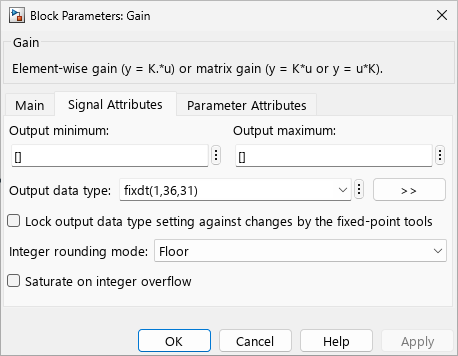Main Content

## Guard Bits

You can eliminate the possibility of overflow by appending the appropriate number of guard bits to a binary word.

For a two's complement signed value, the guard bits are filled with either 0's or 1's depending on the value of the most significant bit (MSB). This is called sign extension. For example, consider a 4-bit two's complement number with value 1011. If this number is extended in range to 7 bits with sign extension, then the number becomes 1111101 and the value remains the same.

The Simulink® software supports guard bits only for fractional data types. For both signed and unsigned fractionals, the guard bits lie to the left of the default binary point. For example, by setting Output data type to `sfrac(36,4)`, you specify a 36–bit signed fractional data type with 4 guard bits (total word size is 40 bits).## SupportGet trial now